### ISLOGICAL Function Examples – Excel, VBA, & Google Sheets

This tutorial demonstrates how to use the Excel ISLOGICAL Function in Excel to test if a cell is a logical value.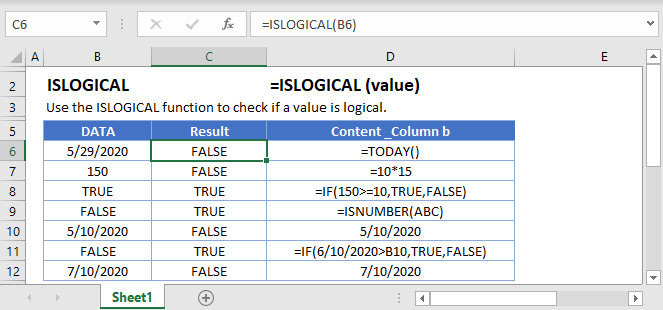## ISLOGICAL Function Overview

The ISLOGICAL Function Test if cell is logical (TRUE or FALSE). Returns TRUE or FALSE.

To use the ISLOGICAL Excel Worksheet Function, select a cell and type: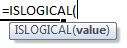(Notice how the formula inputs appear)

### ISLOGICAL Function Syntax and Inputs:

`=ISLOGICAL(VALUE)`

value – The test value

AutoMacro - VBA Code Generator

## How to use the ISLOGICAL Function

The ISLOGICAL Function tests if a cell contains a logical expression (TRUE or FALSE). If the cell contains a logical expression it returns TRUE, otherwise it returns FALSE.

`=ISLOGICAL(A2)`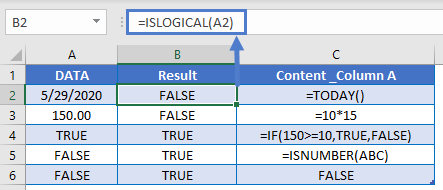### If Is Logical

Usually, after performing a logical test, you will want to do something based on the result of that test. The IF Function can be used to perform different actions if a statement is evaluated as TRUE or FALSE. Let’s use it with the ISLOGICAL Function.

`=IF(ISLOGICAL(A2),"logical","not logical")`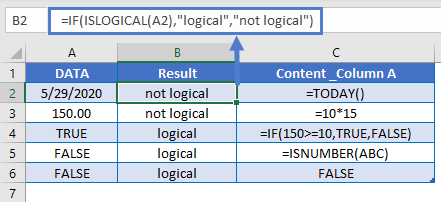### If Is Not Logical

The NOT Function inverts logical results. It changes TRUE to FALSE and FALSE to TRUE. Here we can use that with the IF Function:

`=IF(NOT(ISLOGICAL(A2)),"not logical", "logical")`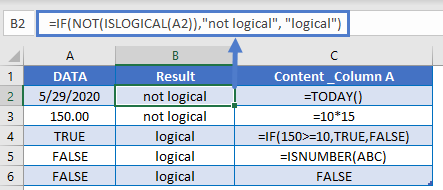### Other Logical Functions

Excel / Google Sheets contain many other logical functions to perform other logical tests. Here is a list:

IF / IS Functions
iferror
iserror
isna
iserr
isblank
isnumber
istext
isnontext
isformula
islogical
isref
iseven
isodd

## ISLOGICAL in Google Sheets

The ISLOGICAL Function works exactly the same in Google Sheets as in Excel: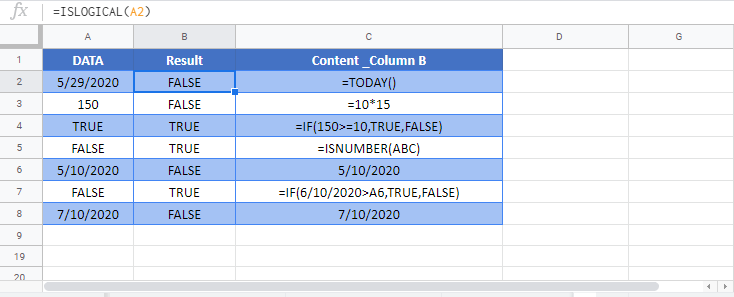## ISLOGICAL Examples in VBA

You can also use the ISLOGICAL function in VBA. Type:
`application.worksheetfunction.islogical(value)`
For the function arguments (value, etc.), you can either enter them directly into the function, or define variables to use instead.

Return to the List of all Functions in Excel

## Excel Practice Worksheet

Practice Excel functions and formulas with our 100% free practice worksheets!

• Automatically Graded Exercises
• Learn Excel, Inside Excel!